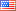Question-and-Answer Resource for the Building Energy Modeling Community
Get s tarted with the Help page

# Revision history [back]

### Best practice for determining if two Surfaces intersect?

Hi all,

I'm looking to find the best way to use the OpenStudio SDK to check if two surfaces intersect. Rather than write my own code to do this, I assumed there's likely something packaged into the SDK to help me do this.

Ideally, the code would look something like.

#Made up function 'intersectsWith'
surfaces_intersect = surface1.intersectsWith(surface2)

if surfaces_intersect
#DO SOMETHING
end


Where

• surface1,surface2 are OpenStudio::Model::Surface
• surfaces_intersect = a boolean that determines if surface1 and surface2 intersect

I've looked into the computeIntersection for Surface found here but am unsure if this is path would be the most elegant way to do this.

For context, I'm trying to dynamically determine the perimeter of a floor surface exposed to ambient to set them for a FFactorGroundFloorConstruction. I haven't seen any way to easily do this in OpenStudio. Here is my approach:

1. Identify if floor with outside boundary condition equal to Ground. If so, flag as a floor requiring a FFactorGroundFloorConstruction
2. Identify all walls in this floor's space. If the wall's outside boundary condition is Outdoors AND it intersects the floor of interest, find it's projection on the x, y plane (i.e. the length of floor that is exposed to perimeter along this wall).
3. Add these perimeters for the floor to ultimately arrive at the exposed perimeter.

I cannot complete step two as I do not have a way to determine if a wall intersects a floor surface.

Any ideas? Thanks!

### Best practice for determining if two Surfaces intersect?

Hi all,

I'm looking to find the best way to use the OpenStudio SDK to check if two surfaces intersect. Rather than write my own code to do this, I assumed there's likely something packaged into the SDK to help me do this.

Ideally, the code would look something like.

#Made up function 'intersectsWith'
surfaces_intersect = surface1.intersectsWith(surface2)

if surfaces_intersect
#DO SOMETHING
end


Where

• surface1,surface2 are OpenStudio::Model::Surface
• surfaces_intersect = a boolean that determines if surface1 and surface2 intersect

I've looked into the computeIntersection for Surface found here but am unsure if this is path would be the most elegant way to do this.

For context, I'm trying to dynamically determine the perimeter of a floor surface exposed to ambient to set them for a FFactorGroundFloorConstruction. I haven't seen any way to easily do this in OpenStudio. Here is my approach:

1. Identify if floor with outside boundary condition equal to Ground. If so, flag as a floor requiring a FFactorGroundFloorConstruction
2. Identify all walls in this floor's space. If the wall's outside boundary condition is Outdoors AND it intersects the floor of interest, find it's projection on the x, y plane (i.e. the length of floor that is exposed to perimeter along this wall). NOTE: This assumes a wall only touches 1 floor; this might not always be true.
3. Add these perimeters for the floor to ultimately arrive at the exposed perimeter.

I cannot complete step two as I do not have a way to determine if a wall intersects a floor surface.

Any ideas? Thanks!3 retagged__AmirRoth__4388 ●5 ●16 http://bleedinggreenna...

### Best practice for determining if two Surfaces intersect?

Hi all,

I'm looking to find the best way to use the OpenStudio SDK to check if two surfaces intersect. Rather than write my own code to do this, I assumed there's likely something packaged into the SDK to help me do this.

Ideally, the code would look something like.

#Made up function 'intersectsWith'
surfaces_intersect = surface1.intersectsWith(surface2)

if surfaces_intersect
#DO SOMETHING
end


Where

• surface1,surface2 are OpenStudio::Model::Surface
• surfaces_intersect = a boolean that determines if surface1 and surface2 intersect

I've looked into the computeIntersection for Surface found here but am unsure if this is path would be the most elegant way to do this.

For context, I'm trying to dynamically determine the perimeter of a floor surface exposed to ambient to set them for a FFactorGroundFloorConstruction. I haven't seen any way to easily do this in OpenStudio. Here is my approach:

1. Identify if floor with outside boundary condition equal to Ground. If so, flag as a floor requiring a FFactorGroundFloorConstruction
2. Identify all walls in this floor's space. If the wall's outside boundary condition is Outdoors AND it intersects the floor of interest, find it's projection on the x, y plane (i.e. the length of floor that is exposed to perimeter along this wall). NOTE: This assumes a wall only touches 1 floor; this might not always be true.
3. Add these perimeters for the floor to ultimately arrive at the exposed perimeter.

I cannot complete step two as I do not have a way to determine if a wall intersects a floor surface.

Any ideas? Thanks!